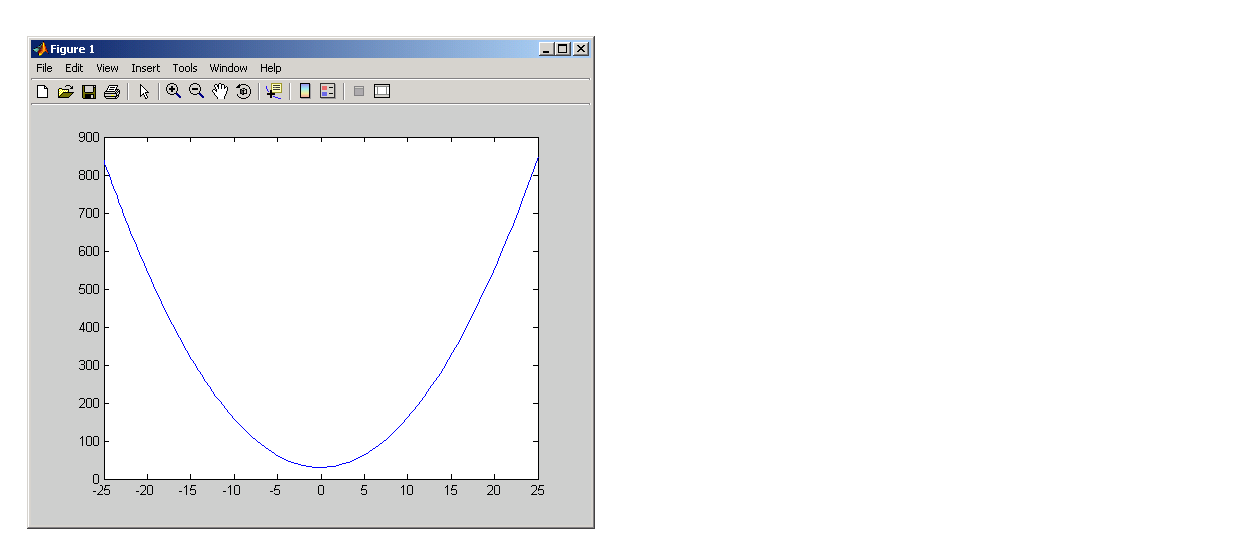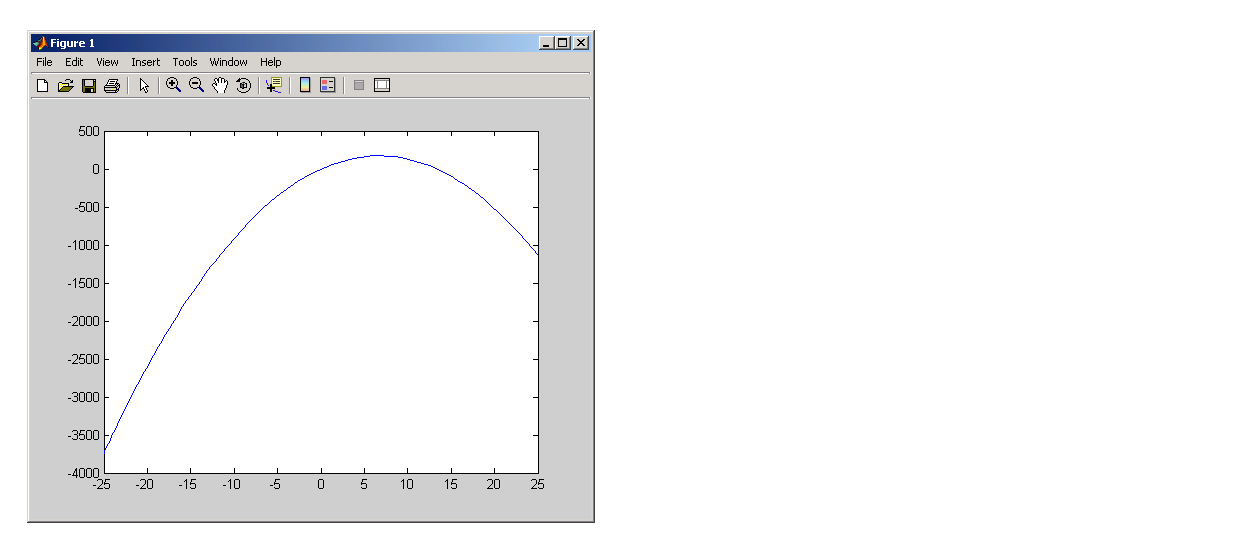ProgrammingVariables Used in the Expression

Anonymous functions commonly include two types of variables:

• Variables specified in the argument list. These often vary with each function call.
• Variables specified in the body of the expression. MATLAB captures these variables and holds them constant throughout the lifetime of the function handle.

The latter variables must have a value assigned to them at the time you construct an anonymous function that uses them. Upon construction, MATLAB captures the current value for each variable specified in the body of that function. The function will continue to associate this value with the variable even if the value should change in the workspace or go out of scope.

The fact that MATLAB captures the values of these variables when the handle to the anonymous function is constructed enables you to execute an anonymous function from anywhere in the MATLAB environment, even outside the scope in which its variables were originally defined. But it also means that to supply new values for any variables specified within the expression, you must reconstruct the function handle.

Changing Variables Used in an Anonymous Function

The second statement shown below constructs a function handle for an anonymous function called `parabola` that uses variables `a`, `b`, and `c` in the expression. Passing the function handle to the MATLAB `fplot` function plots it out using the initial values for these variables:

• ```a = 1.3;   b = .2;   c = 30;
parabola = @(x) a*x.^2 + b*x + c;
fplot(parabola, [-25 25])```

If you change the three variables in the workspace and replot the figure, the parabola remains unchanged because the `parabola` function is still using the initial values of `a`, `b`, and `c`:

• ```a = -3.9;   b = 52;   c = 0;
fplot(parabola, [-25 25])```

To get the function to use the new values, you need to reconstruct the function handle, causing MATLAB to capture the updated variables. Replot using the new construct, and this time the parabola takes on the new values:

• ```a = -3.9;   b = 52;   c = 0;
parabola = @(x) a*x.^2 + b*x + c;
fplot(parabola, [-25 25])```

For the purposes of this example, there is no need to store the handle to the anonymous function in a variable (`parabola`, in this case). You can just construct and pass the handle right within the call to `fplot`. In this way, you update the values of `a`, `b`, and `c` on each call:

• ```fplot(@(x) a*x.^2 + b*x + c, [-25 25])
```Outputs from Anonymous Functions Examples of Anonymous Functions© 1994-2005 The MathWorks, Inc.